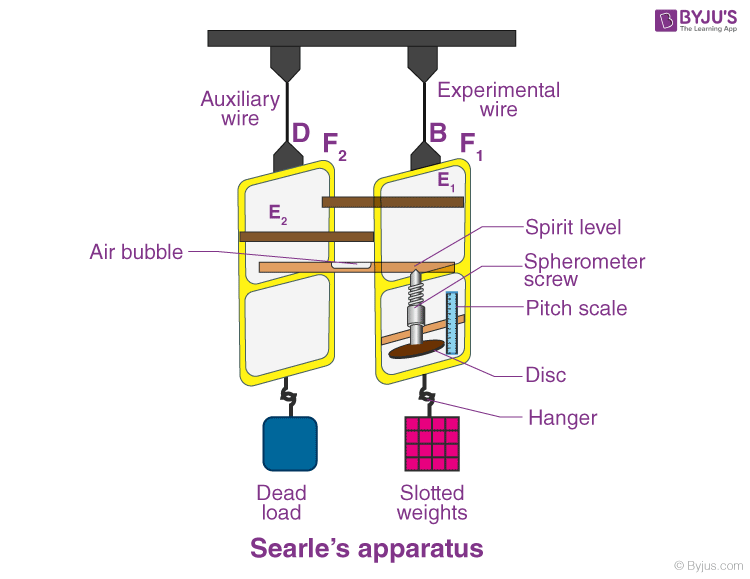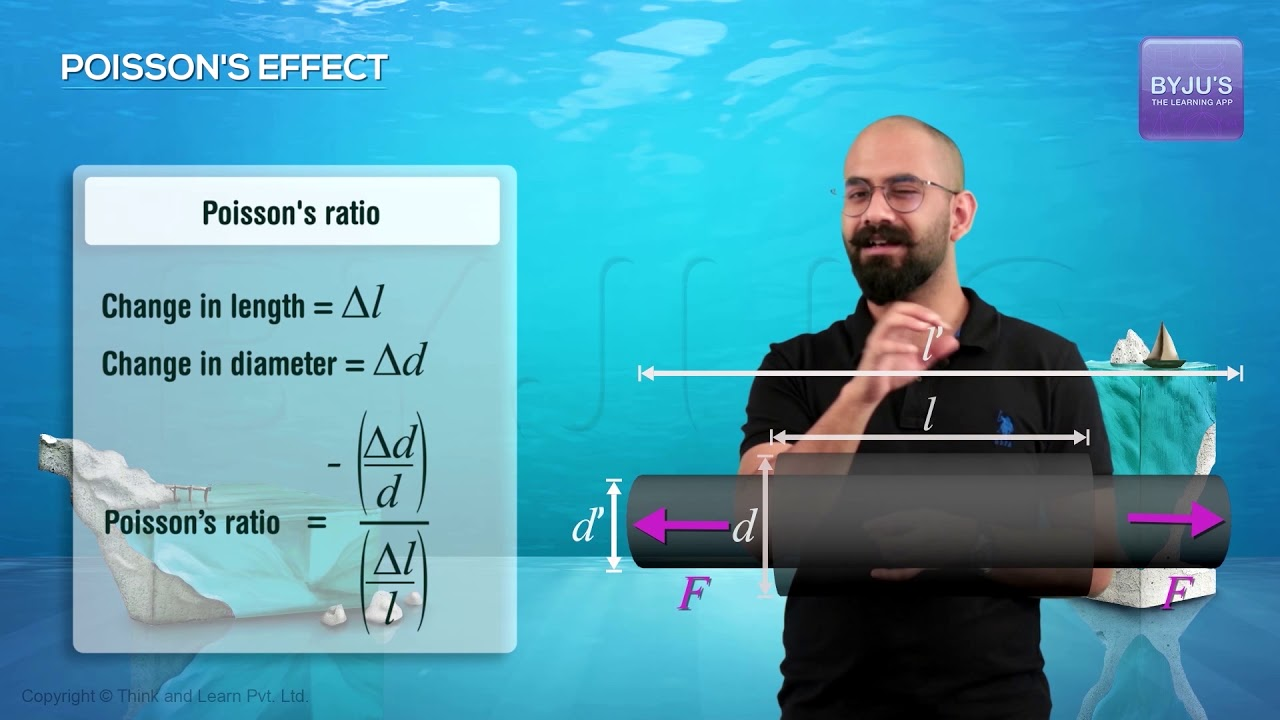# To Determine Young’s Modulus of Elasticity of the Material of a Given Wire

Searle’s apparatus is used for the measurement of Young’s modulus. It consists of two equal-length wires that are attached to a rigid support. To understand how Searle’s apparatus is used to determine Young’s modulus of elasticity of the material of a given wire, read the experiment below.

## Aim

To determine Young’s modulus of elasticity of the material of a given wire.

## Materials Required

1. Searle’s apparatus
2. Two long steel wires of the same length and diameter
3. A metre scale
4. A screw gauge
5. Eight 0.5 kg slotted weights
6. 1 kg hanger

## Theory

The normal stress for a wire with length L and radius r is loaded with weight Mg where l is the increase in length, then normal stress is given as:

$$\begin{array}{l}Normal\,stress = \frac{Mg}{\pi r^{2}}\end{array}$$

$$\begin{array}{l}Longitudinal\,strain = \frac{l}{L}\end{array}$$

Hence,

$$\begin{array}{l}Young’s\,modulus = \frac{normal\;stress}{longitudinal\;strain}\end{array}$$

$$\begin{array}{l}Y=\frac{\frac{Mg}{\pi r^{2}}}{\frac{l}{L}}\end{array}$$

$$\begin{array}{l}Y=\frac{MgL}{\pi r^{2}l}\end{array}$$

Y can be calculated as the values of L and r are known and l is found by the known Mg value.

## Diagram## Observations

Length of experimental wire AB, L = ….. cm = ……m

Measurement of diameter of the wire

Pitch of the screw gauge (p) = 0.1 cm

No.of divisions on the circular scale = 100

Least count of screw gauge (L.C) = 0.1/100 = 0.001 cm

Zero error of screw gauge (e) = …….cm

Zero error of screw gauge (e) = -e = ……cm

Diameter of experimental wire

 Sl.no Linear scale reading N (cm) Circular scale reading Total reading N+n×(LC)d (cm) No.of divisions on reference line (n) Value n×(LC)(cm) 1. d1= d2= 2. d3= d4= 3. d5= d6= 4. d7= d8= 5. d9= d10=

Measurement for extension of the wire

Breaking stress for steel (from a table), B = ….. Nm-2

Area of a cross-section of a wire, πr2 = ….. cm2 = …….m2

Breaking load = Bπr2 = …. N

$$\begin{array}{l}\frac{B.\pi r^{2}}{9.8}=…..kg\end{array}$$

(∵1 kg = 9.8 N)

1/3rd of breaking load = ….kg

Pitch of spherometer screw, (p) = 0.1 cm

No.of divisions in the disc = 100

Least count of spherometer (LC) = 0.1/100 = 0.001 cm

 Sl.no Load on hanger M (kg) Spherometer screw reading Extension for load 2.5 kg l(cm) Load increasing x(cm) Load decreasing y (cm) Mean z = (x+y)/2 (cm) 1. 0.0 z1= 2. 0.5 z2= 3. 1.0 z3= 4. 1.5 z4= 5. 2.0 z5= 6. 2.5 z6= l1=(z6-z1)= 7. 3.0 z7= l2=(z7-z2)= 8. 3.5 z8= l3=(z8-z3)= 9. 4.0 z9= l4=(z9-z4)=

## Calculations

From table 1

Mean observed diameter of the wire,

$$\begin{array}{l}d_{0}=\frac{d_{1}+d_{2}+…..+d_{10}}{10}=…..cm\end{array}$$

Mean corrected diameter of the wire,

d = (d0 + c) = ……cm = ……m

r = d/2 = ……m

From table 2

Mean extension for 2.5 kg load,

$$\begin{array}{l}l=\frac{l_{1}+l_{2}+l_{3}+l_{4}}{4}=…..cm=….m\end{array}$$

From formula,

$$\begin{array}{l}Y=\frac{MgL}{\pi r^{2}l}=\frac{2.5*9.8*l}{\pi r^{2}*l}Nm^{-2}\end{array}$$

## Result

1. The Young’s modulus for steel as determined by Searle’s apparatus = …….. Nm-2
2. Straight-line graph between load and extension shows that stress ∝ strain. This verifies Hooke’s law.

Percentage error

Actual value of Y for steel =…… Nm-2

The difference in values =……… Nm-2

Percentage error = (difference in values)/(actual value) = …….%

It is within the limits of experimental error.

## Precautions

1. The material, length, and cross-sectional area of both the wires must be the same.
2. The same rigid support should be used as a support for both the wires.
3. Before starting the experiment, kinks should be removed.
4. At different places, the diameter of the wire should be measured.
5. Adding and removing the slotted weight should be done gently.
6. After every addition or removal of weight, wait for two minutes.
7. Increasing or decreasing weights should be done in regular steps.

## Sources of Error

1. The slotted weights might not be of standard weight.
2. The wire used in the experiment may not be of uniform cross-sectional area.

## Viva Questions

Q1. State Hooke’s law.

Ans: Hooke’s law states that when the material has a load within the elastic limit, then the stress is directly proportional to the strain.

Q2. What is Poisson’s ratio?

Ans: Poisson’s ratio is defined as the ratio of lateral strain to longitudinal strain and is denoted by μ.

Q3. What is the yield point?

Ans: Yield point is defined as the stress beyond which the material becomes plastic.

Q4. What is the elastic limit?

Ans: Elastic limit is defined as the maximum limit to which a solid can be stretched without any permanent alteration of shape or size.

Q5. What is a lateral strain?

Ans: Lateral strain is defined as the ratio of axial deformation to the original length of the body.